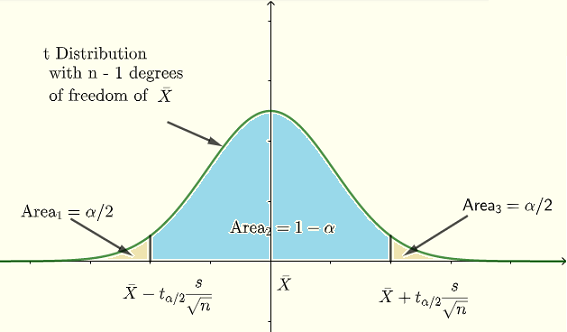# Confidence Interval Using t Distribution Calculator

  

An online and easy to use calculator that calculates the confidence interval with a certain percentage, using the t distribution, is presented.
An online calculator that calculates the confidence interval using normal distribution calculator is included.

## Definition of Confidence Interval for the t Distribution

For a sample of size $$n$$ with standard deviation $$s$$, we define a $$(1-\alpha)100\%$$ confidence interval for $$\mu$$ as $\bar X \pm t_{\alpha/2} \dfrac{s}{\sqrt n}$ We say that we are $$(1-\alpha)100\%$$ confident that the mean $$\mu$$ of the population is within the interval $\left[\bar X - t_{\alpha/2} \dfrac{s}{\sqrt n} \quad , \quad \bar X + t_{\alpha/2} \dfrac{s}{\sqrt n} \right]$.
where $$t_{\alpha/2}$$ is the value of the t distribution with $$n - 1$$ degrees of freedom such that the areas to the left and to the right are equal to $$\alpha/2$$ as shown in the graph below.
The graphical meaning of an interval of confidence is shown below.The above definition is used when the standard deviation of the population $$P$$ is NOT known but the sample standard deviation $$s$$ is known and/or the sample size is not large $$(n \lt 30)$$.

## Confidence Interval Calculator

Enter the sample size $$n$$ as a positive integer, the sample mean $$\bar X$$, the sample standard deviation $$s$$ as a positive real number and the level of confidence (percentage) as a positive real number greater than $$0$$ and smaller than $$100$$.

Sample Size: $$n$$ =
Sample Mean: $$\bar X$$ =
Sample Standard Deviation: $$s$$ =
Confidence Level = $$\%$$

Decimal Places =